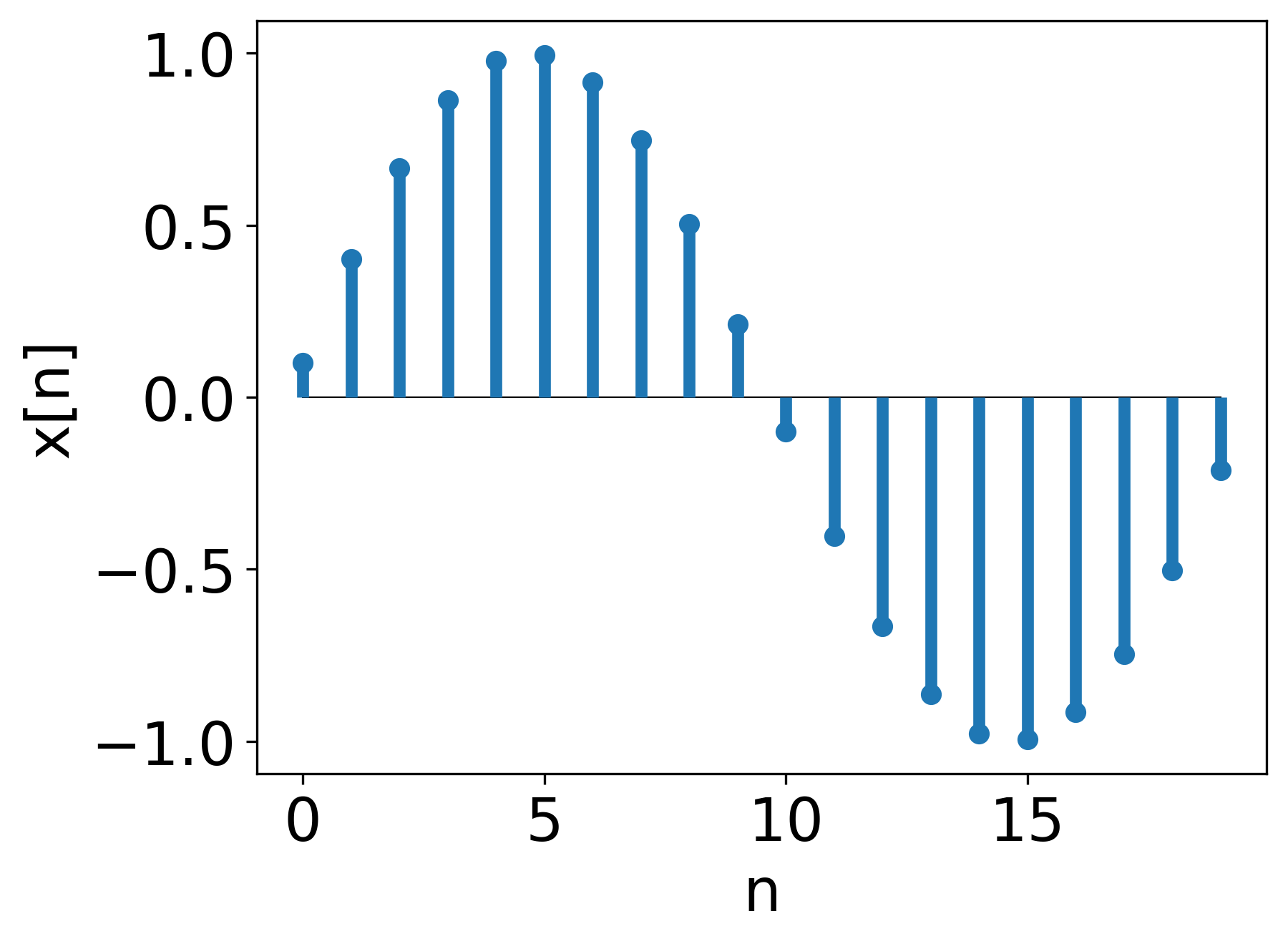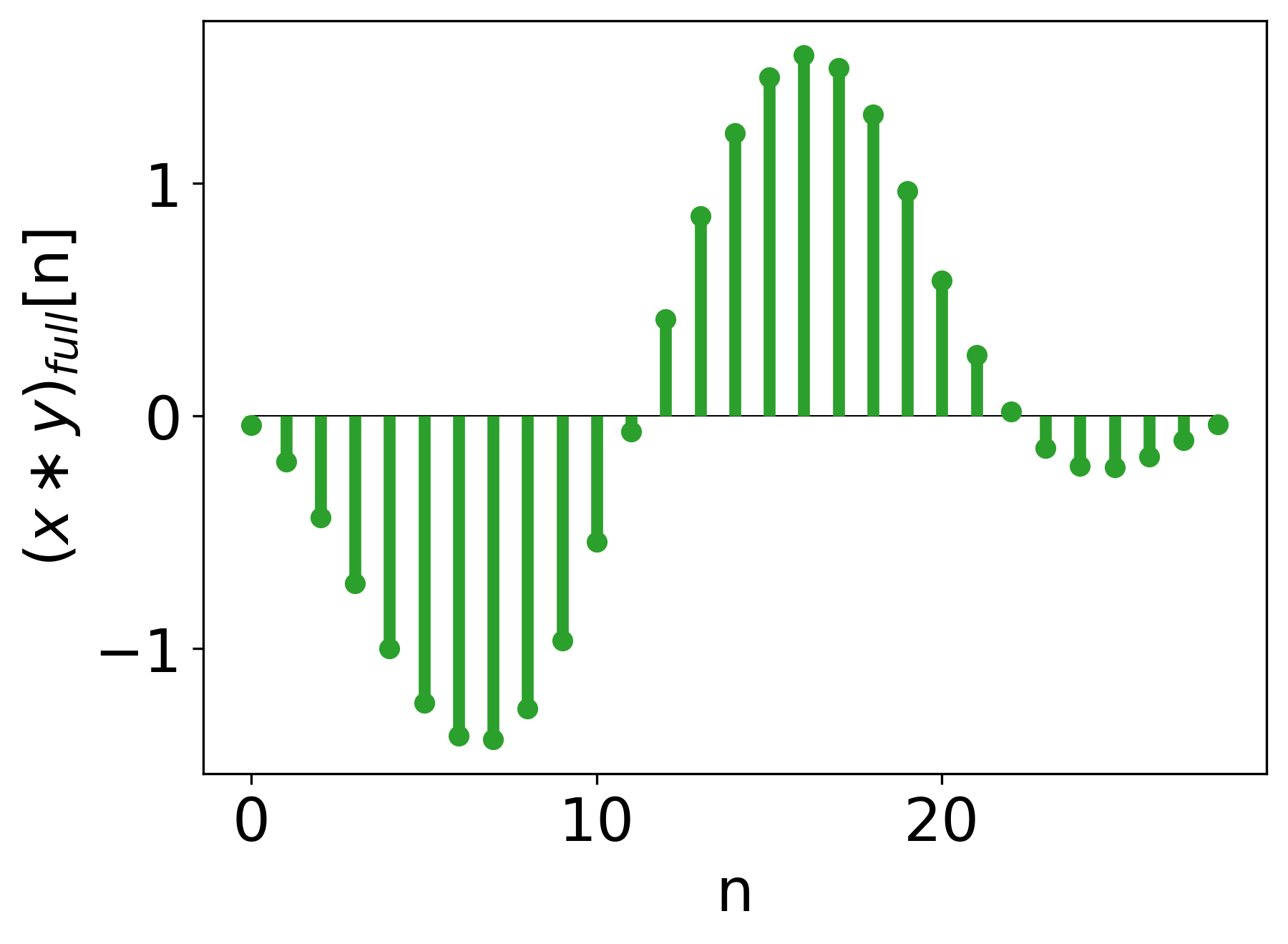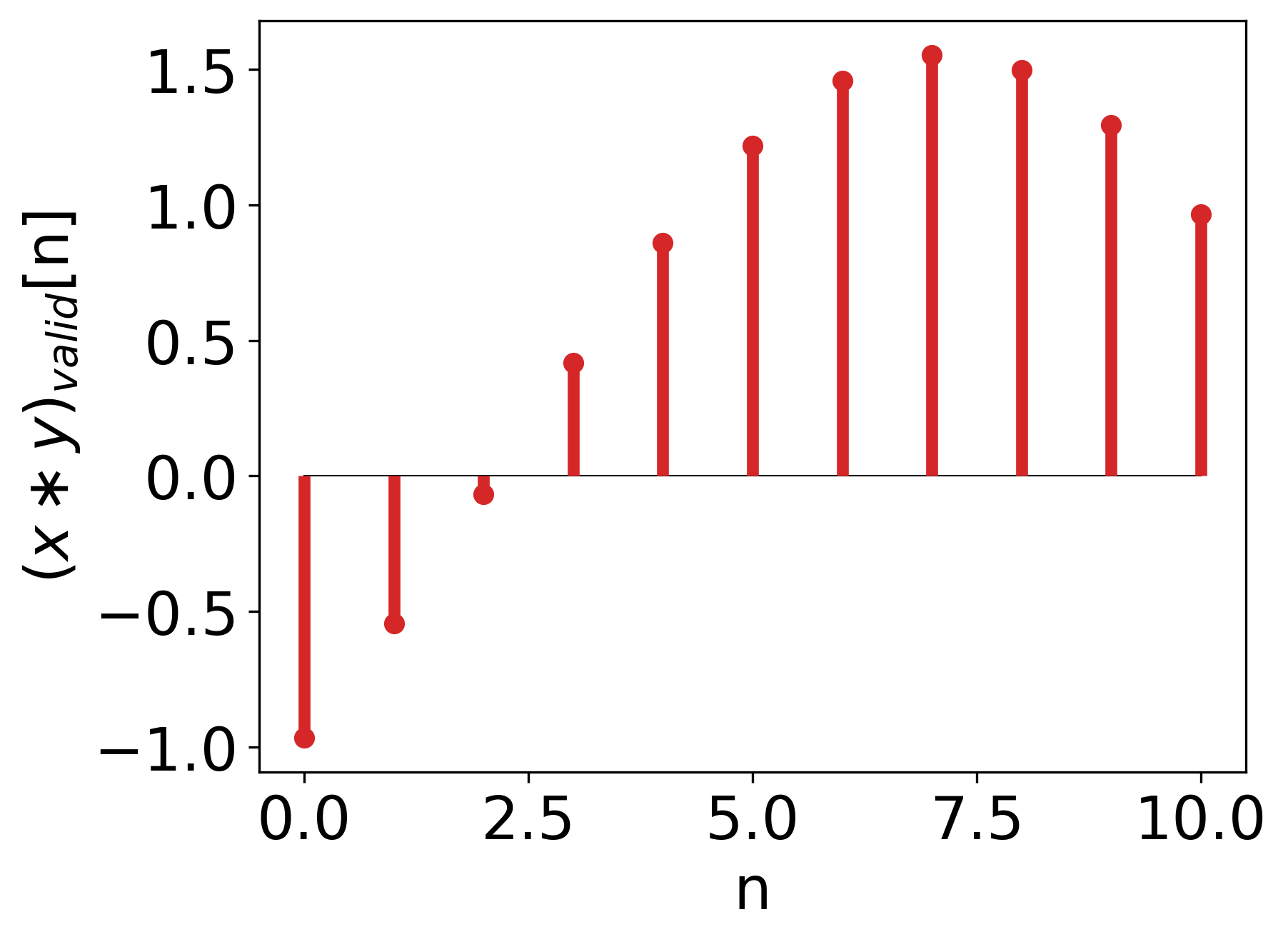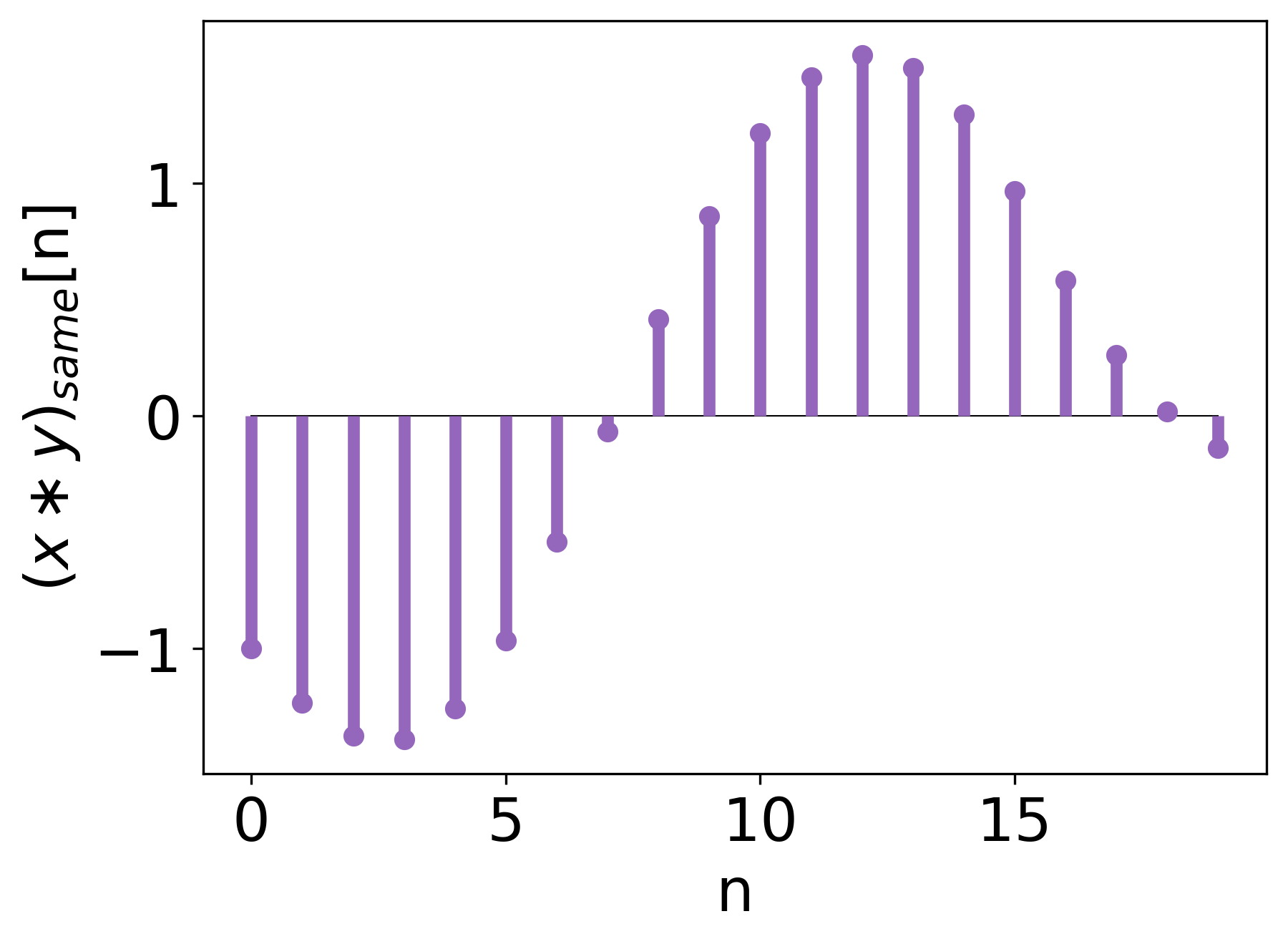How to compute convolution using numerical software libraries?

### The Convolution Series

Most often we won’t be implementing convolution every time we need to use it. Therefore, it is important to know functions from numerical software libraries we can use.

The most popular implementation of the convolution are conv from Matlab, convolve from NumPy, and convolve from SciPy. I won’t be describing any C/C++ convolution implementations here.

## 3 Modes of Convolution

Before we dive into the specific functions, it is important to understand 3 different ‘modes’ the convolution can be calculated with.

We will observe their effect using the following signals: $x[n]$Figure 1. $x[n]$.

and $y[n]$Figure 2. $y[n]$.

### Full

‘Full’ is the mathematical implementation of convolution. Having signals of length $M$ and $N$, the ‘full’ mode returns a signal of length $M + N - 1$. At points where signals do not overlap, they are padded with zeros.Figure 3. ‘Full’ mode of the convolution.

This is the default option for Matlab, NumPy, and SciPy.

### Valid

‘Valid’ mode does not use zero padding at all. The output is calculated only at positions where signals overlap completely. The result is a very short vector of length $\max(M, N) - \min(M, N) + 1$.Figure 4. ‘Valid’ mode of the convolution.

Note that using this mode of convolution shrinks the output signal with each application .

### Same

‘Same’ acts as an intermediate level between ‘full’ and ‘valid’; it crops the middle part out of the ‘full’ mode. Its length is equal to the length of the longer signal (NumPy, SciPy) or the first signal given (Matlab). This approach comes in handy when we want to keep the size of the convolution output constant.Figure 5. ‘Same’ mode of the convolution.

## Convolution Functions

Knowing the 3 modes, we can present now convolution functions of different numerical software libraries.

### NumPy

numpy.convolve has the following signature

output = numpy.convolve(x, y, mode='full')


x and y are 1-D-arrays and mode is a string containing the convolution mode name.

### SciPy

scipy.signal.convolve has the following signature

output = scipy.signal.convolve(x, y, mode='full', method='auto')


x and y are N-D-arrays, mode is a string containing the convolution mode name, and method can be direct (evaluation according to the convolution definition), fft (equivalent to the usage of scipy.signal.fftconvolve, i.e., the fast convolution algorithm), or auto (let the software decide).

FFT convolution (fast convolution) is recommended for long signals of similar size.

SciPy has another convolution function, namely, oaconvolve. It lets the user pick the axes to compute convolution over. It uses the overlap-add scheme and, thus, is recommended for long signals of significanlty different sizes.

oaconvolve and fftconvolve have the same signature: they take two multidimensional input signals, mode argument, and axes argument to compute the convolution over (an integer, an array, or None to use all axes).

scipy.signal.fftconvolve(x, y, mode='full', axes=None)
scipy.signal.oaconvolve(x, y, mode='full', axes=None)


### Matlab

Matlab’s conv has the following signature

output = conv(x, y, shape) % shape is 'full' if not explicitly given


x and y are 1-D, row or column vectors. For 2-D convolution, one may use conv2 function, and for N-D convolution, there is convn function.

## Summary

In this article, we have discussed 3 modes of convolution: full, valid, and same and the implementations of convolution in NumPy, SciPy, and Matlab.

Check out the references below for more details.

## Bibliography

 I. Goodfellow, Y. Bengio, A. Courville Deep learning, MIT Press, 2016, https://www.deeplearningbook.org/.